Скачать презентацию A two stage raw material stock optimization model

c7f04030bb60ee946a706f7be066af6c.ppt

• Количество слайдов: 30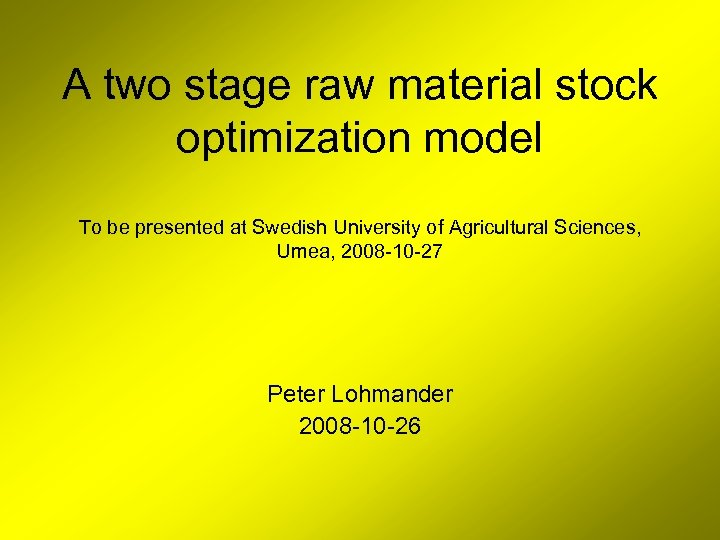A two stage raw material stock optimization model To be presented at Swedish University of Agricultural Sciences, Umea, 2008 -10 -27 Peter Lohmander 2008 -10 -26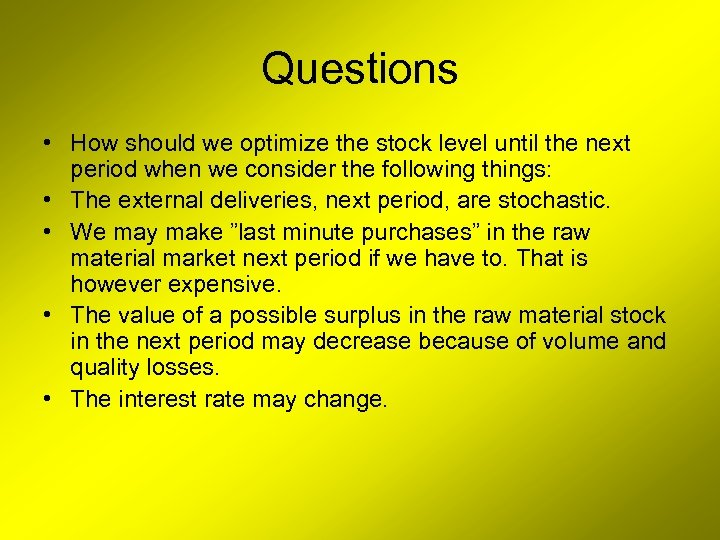Questions • How should we optimize the stock level until the next period when we consider the following things: • The external deliveries, next period, are stochastic. • We may make ”last minute purchases” in the raw material market next period if we have to. That is however expensive. • The value of a possible surplus in the raw material stock in the next period may decrease because of volume and quality losses. • The interest rate may change.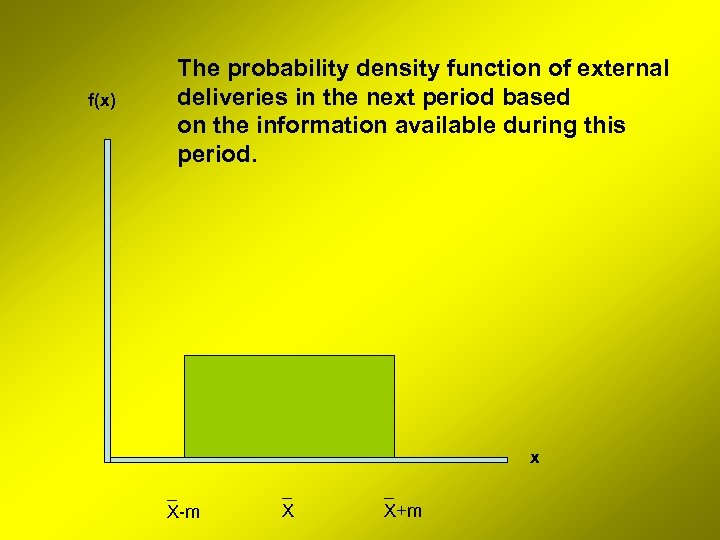f(x) The probability density function of external deliveries in the next period based on the information available during this period. x _ X-m _ X+m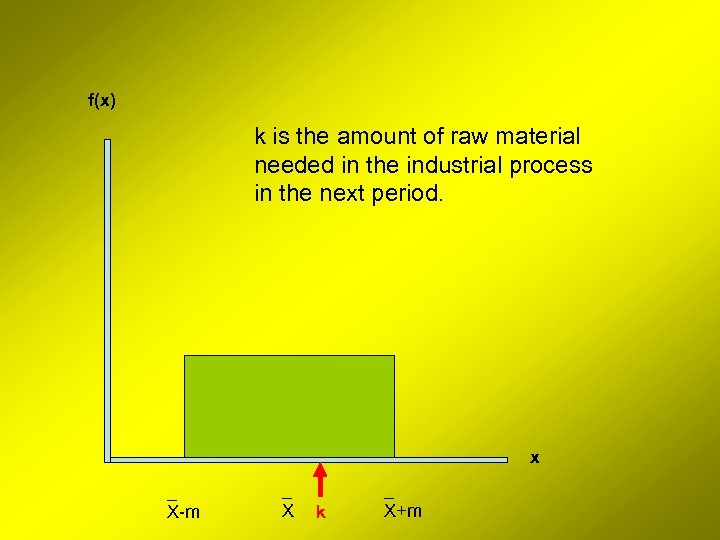f(x) k is the amount of raw material needed in the industrial process in the next period. x _ X-m _ X k _ X+m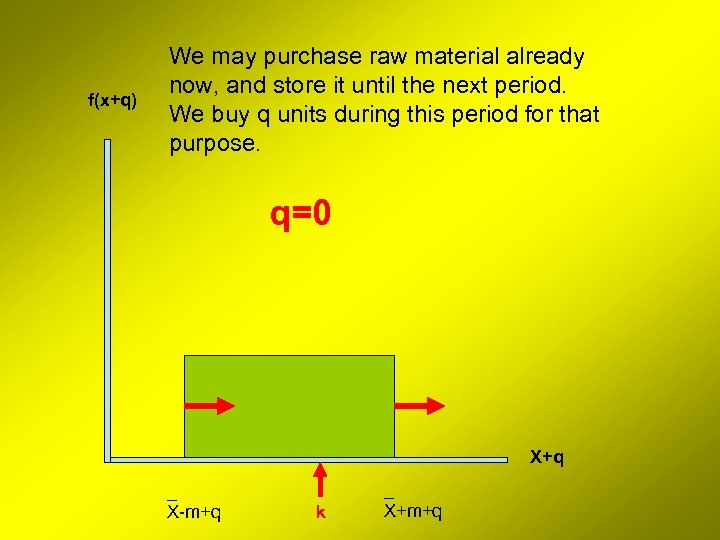f(x+q) We may purchase raw material already now, and store it until the next period. We buy q units during this period for that purpose. q=0 X+q _ X-m+q k _ X+m+q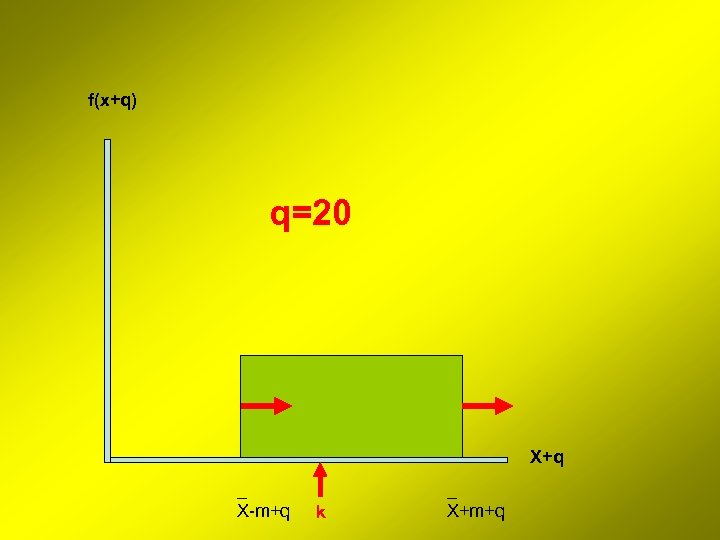f(x+q) q=20 X+q _ X-m+q k _ X+m+q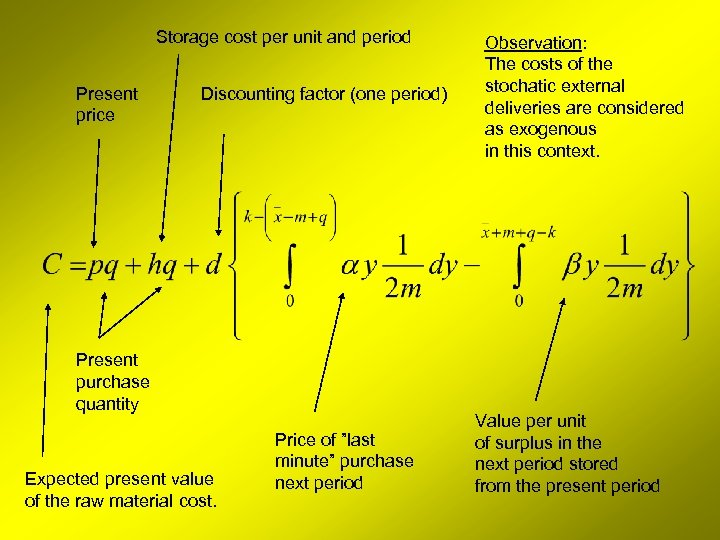Storage cost per unit and period Present price Discounting factor (one period) Present purchase quantity Expected present value of the raw material cost. Price of ”last minute” purchase next period Observation: The costs of the stochatic external deliveries are considered as exogenous in this context. Value per unit of surplus in the next period stored from the present period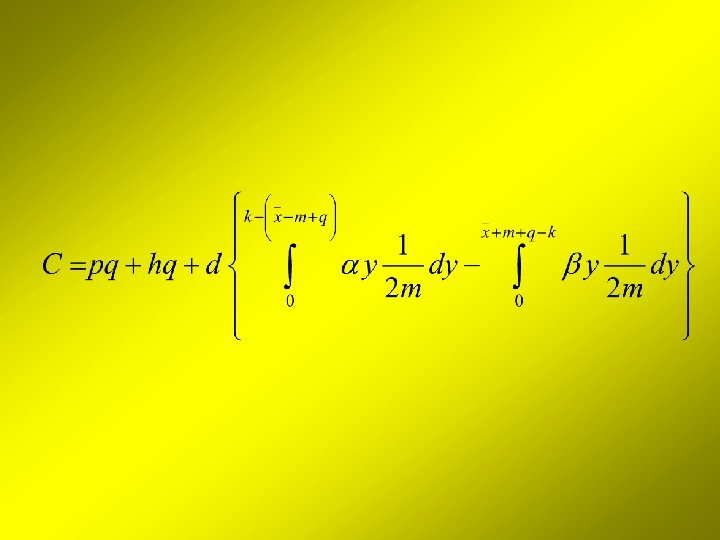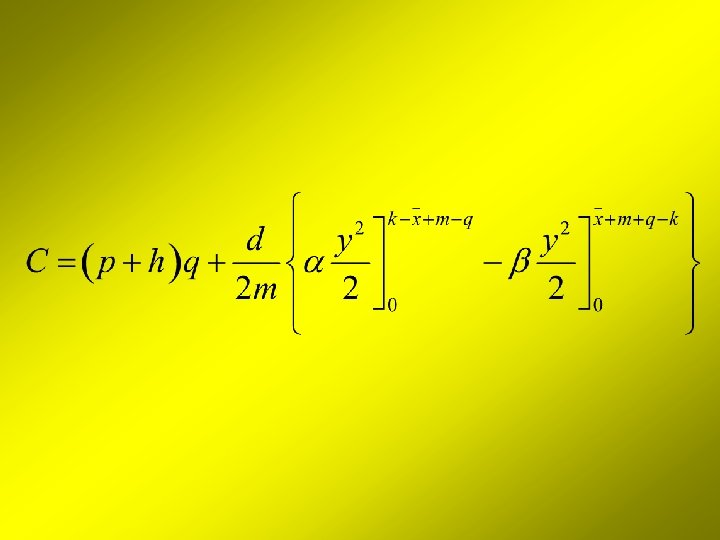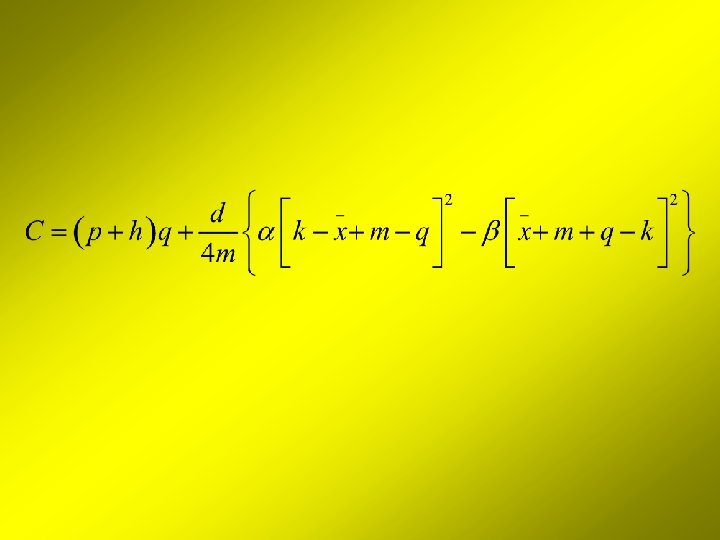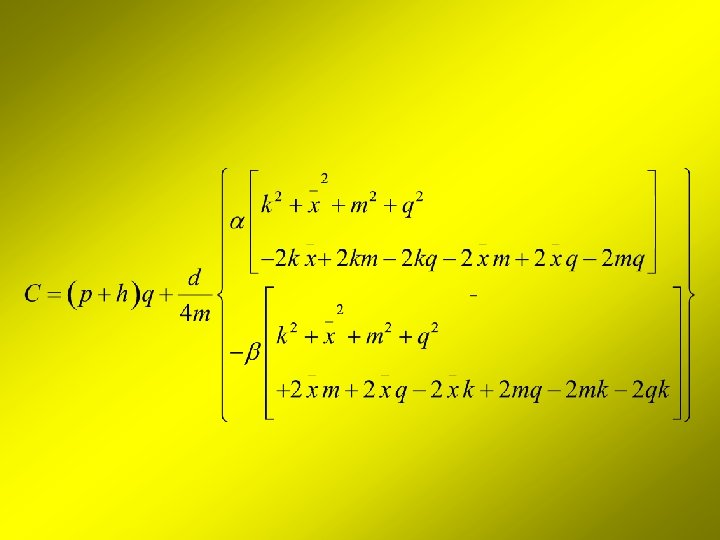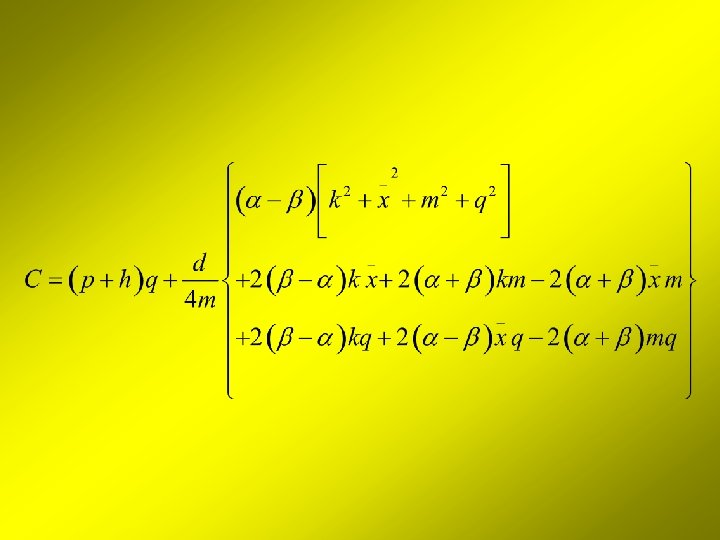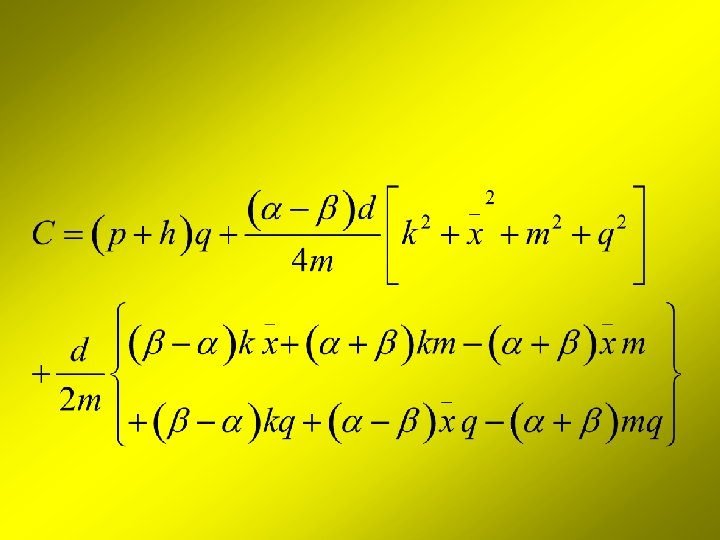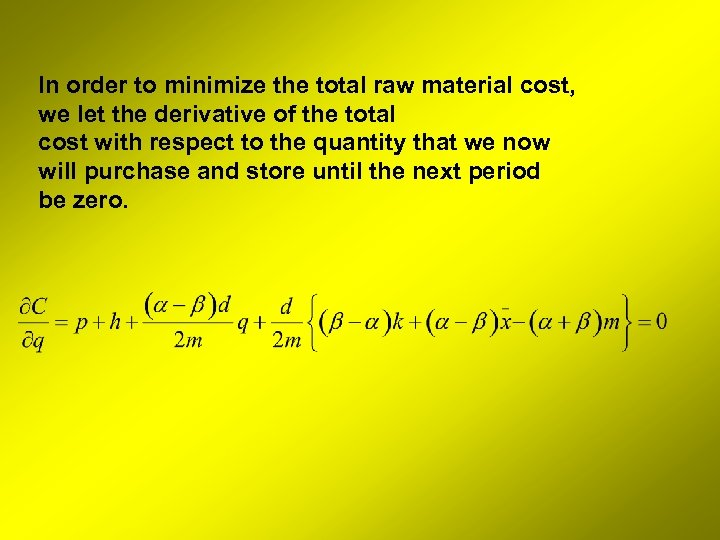In order to minimize the total raw material cost, we let the derivative of the total cost with respect to the quantity that we now will purchase and store until the next period be zero.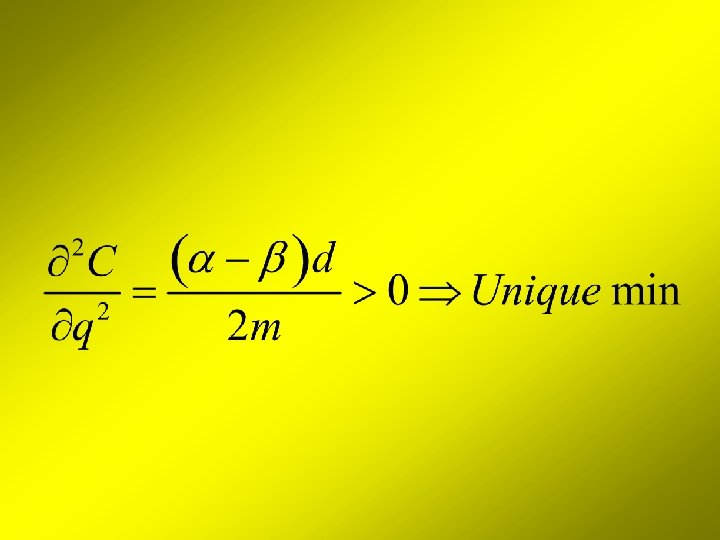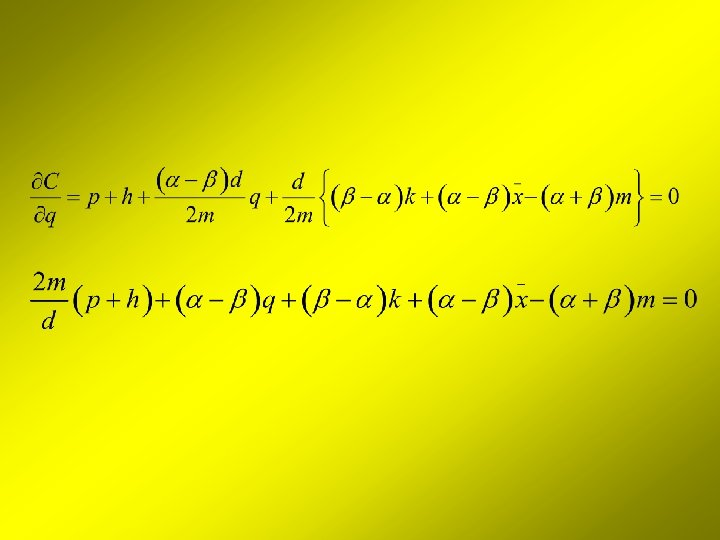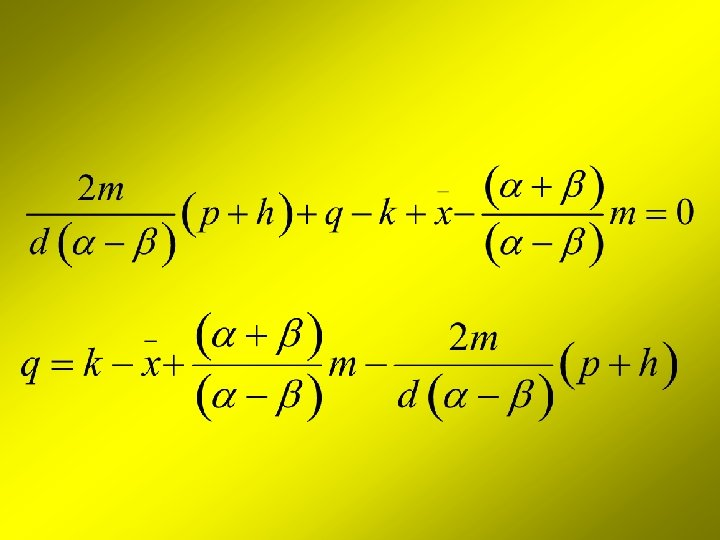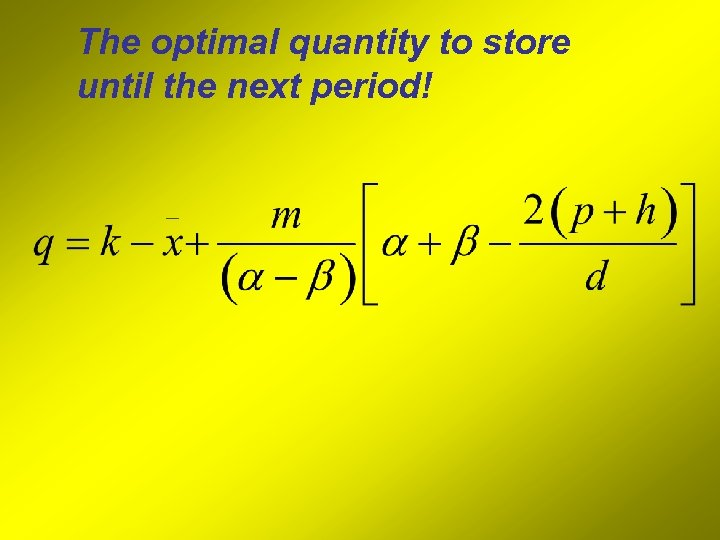The optimal quantity to store until the next period!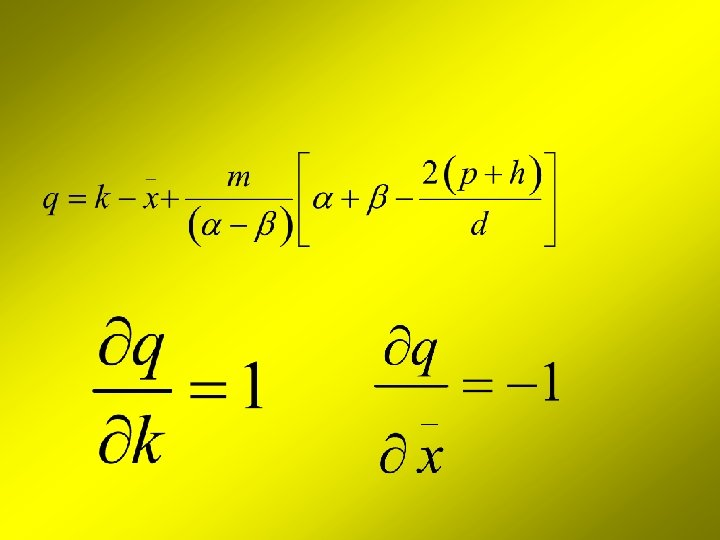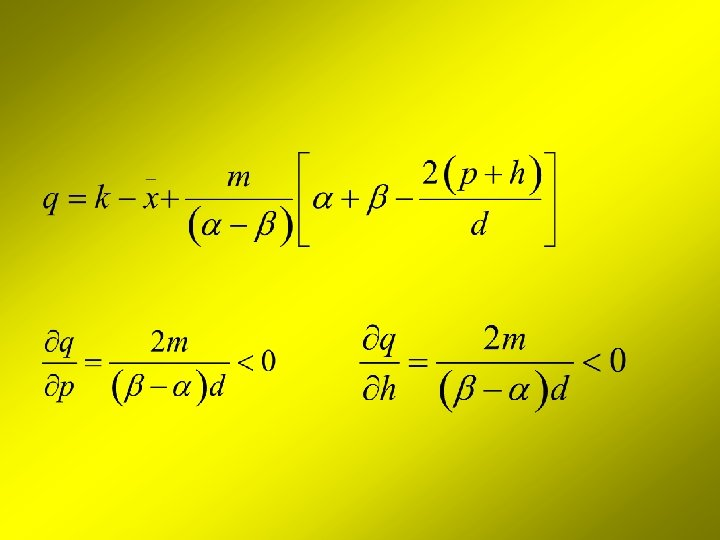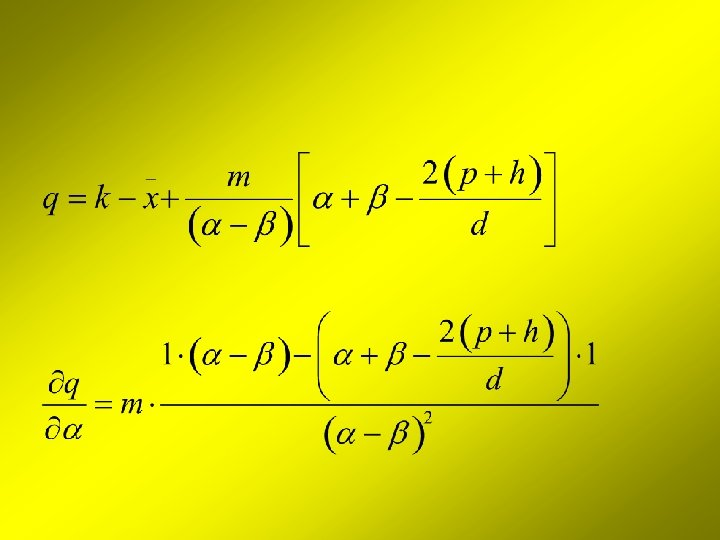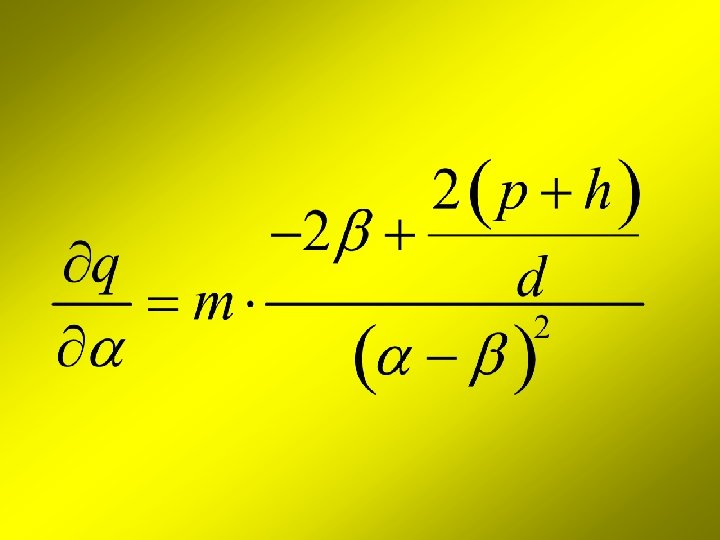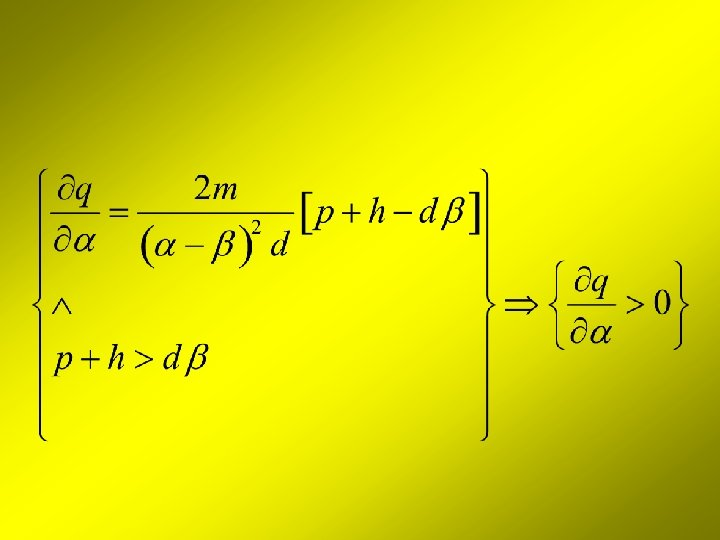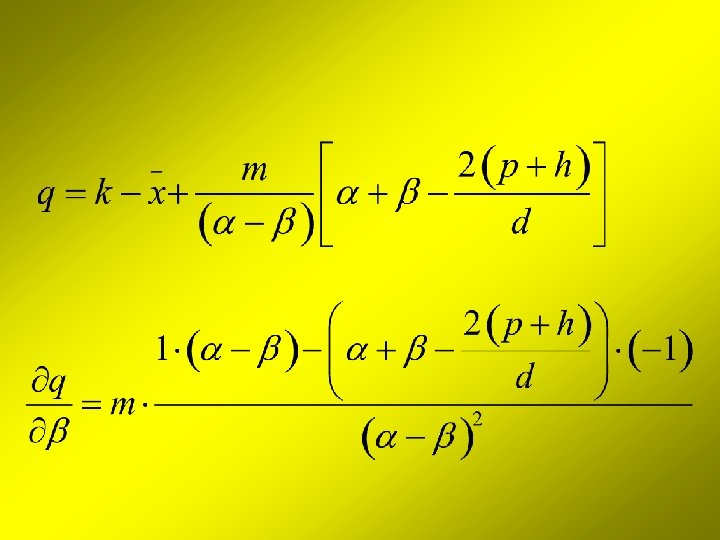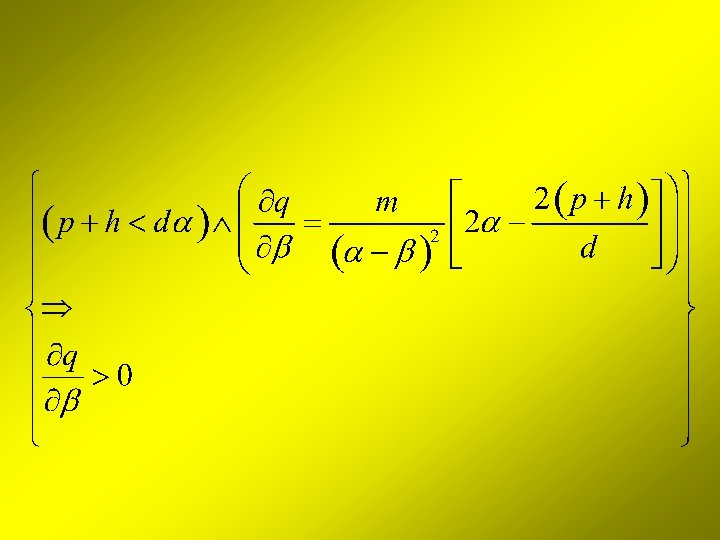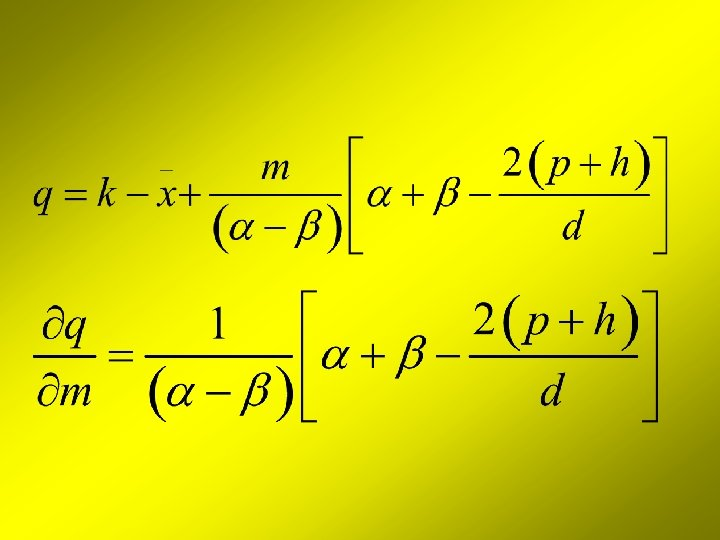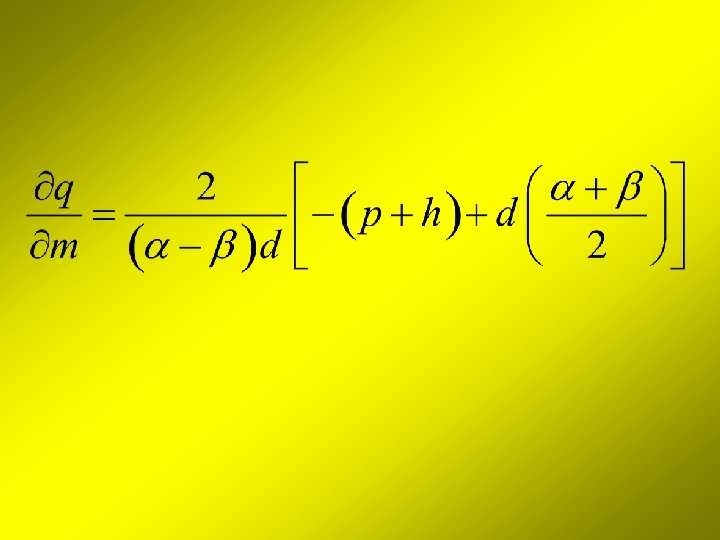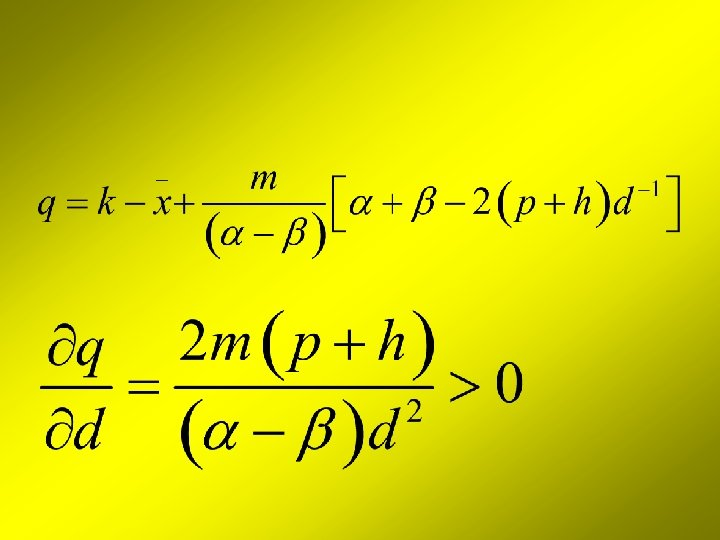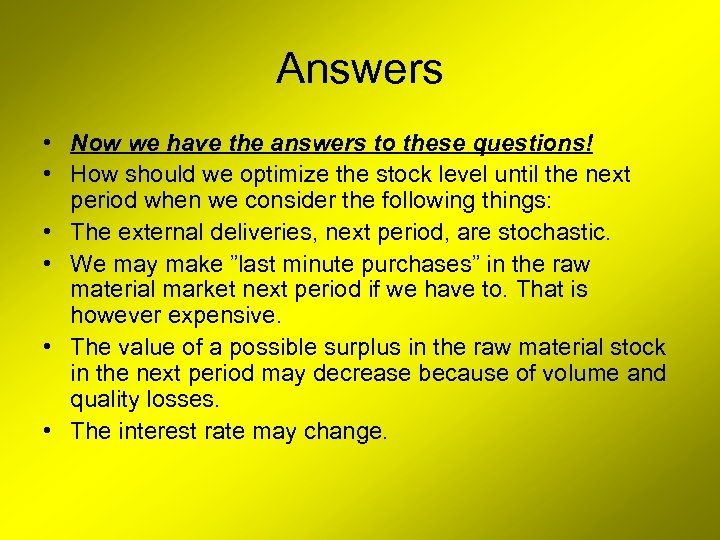Answers • Now we have the answers to these questions! • How should we optimize the stock level until the next period when we consider the following things: • The external deliveries, next period, are stochastic. • We may make ”last minute purchases” in the raw material market next period if we have to. That is however expensive. • The value of a possible surplus in the raw material stock in the next period may decrease because of volume and quality losses. • The interest rate may change.Questions? You are welcome to contact me. Peter Lohmander, Swedish University of Agricultural Sciences, Faculty of Forest Sciences, Dept. of Forest Economics, SE 901 83 Umea, Sweden • Peter. [email protected] slu. se • http: //www. Lohmander. com